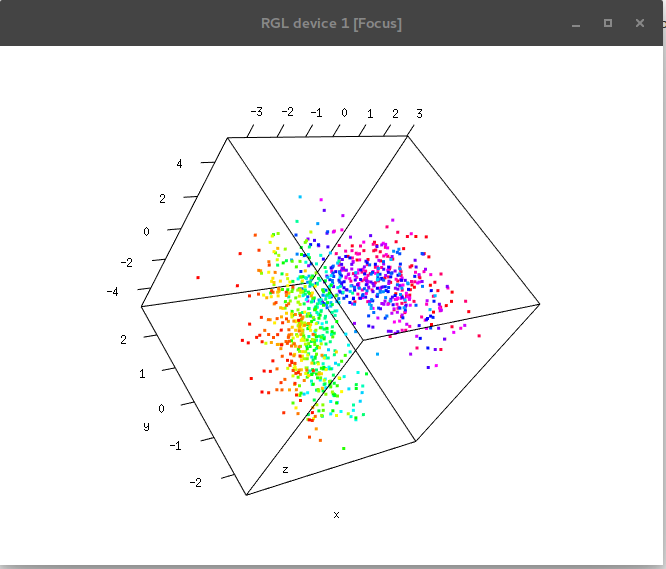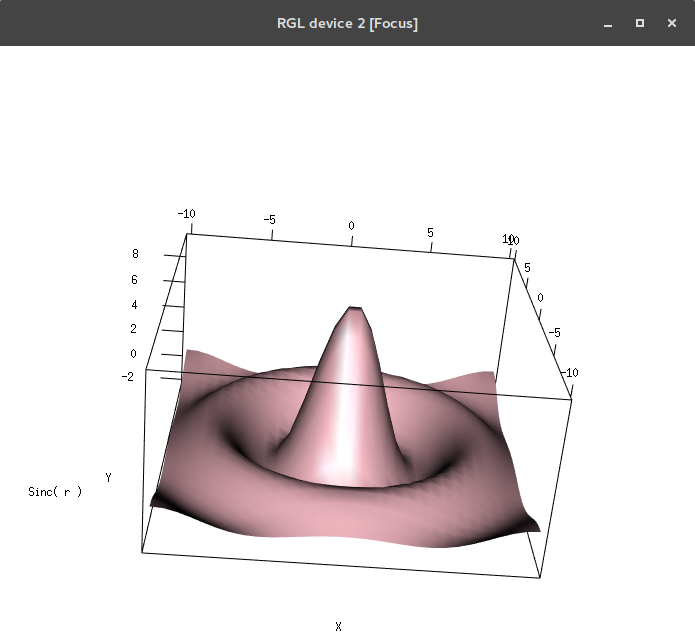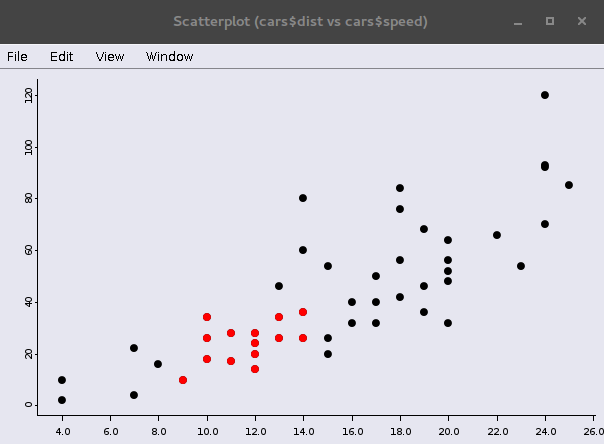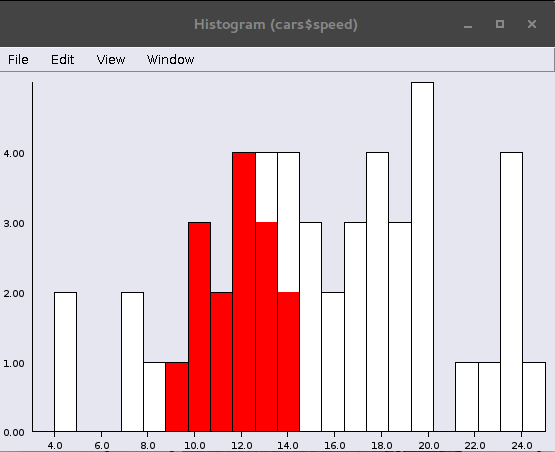# Introduction to interactive graphics

Nathalie Vialaneix and Sébastien Déjean
12 octobre 2018

## Interactivity

### Interactivity in data analysis

Why?

• Deeper exploration of data than static plots
• Immediate feedback on how data/figures/results change when inputs are modified
• User becomes an active participant in the analysis
• Exploratory analysis, teaching, reporting

### An overview of some solutions

Evaluation of the different solution on http://ouzor.github.io/blog/2014/11/21/interactive-visualizations.html (maybe a bit outdated).

## rgl

library(rgl)
x <- sort(rnorm(1000))
y <- rnorm(1000)
z <- rnorm(1000) + atan2(x, y)
plot3d(x, y, z, col = rainbow(1000))• Open a new graphic device outside R or Rstudio

• Zoom and rotation using the mouse

## rgl: surface plots

x <- seq(-10, 10, length = 30)
y <- x
f <- function(x, y) { r <- sqrt(x^2 + y^2); 10 * sin(r)/r }
z <- outer(x, y, f)
z[is.na(z)] <- 1
open3d()
bg3d("white")
material3d(col = "black")
persp3d(x, y, z, aspect = c(1, 1, 0.5), col = "lightpink",
xlab = "X", ylab = "Y", zlab = "Sinc( r )")• The Obligatory Mathematical surface!

• Many other possibilities: cube3d, decorated3d, ellipse3d

## iplots: scatter plot and histogram

library(iplots)
data(cars)
iplot(cars$speed, cars$dist, ptDiam=8)
ihist(cars$speed)• Interactivity between several graphical devices opened outside R or Rstudio • Points selected on one plot are highlighted on the others ## iplots: barplot and mosaicplot library(MASS) data(Cars93) imosaic(Cars93$AirBags, Cars93$DriveTrain) ibar(Cars93$AirBags)
ibar(Cars93\$DriveTrain)